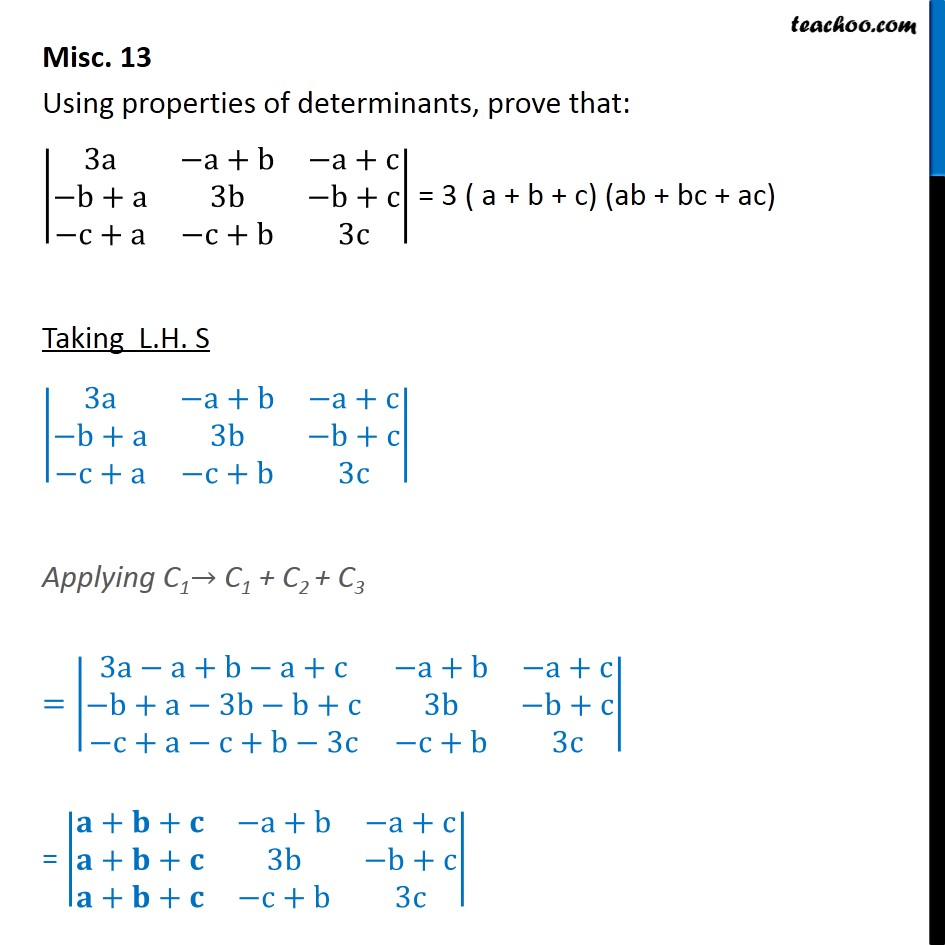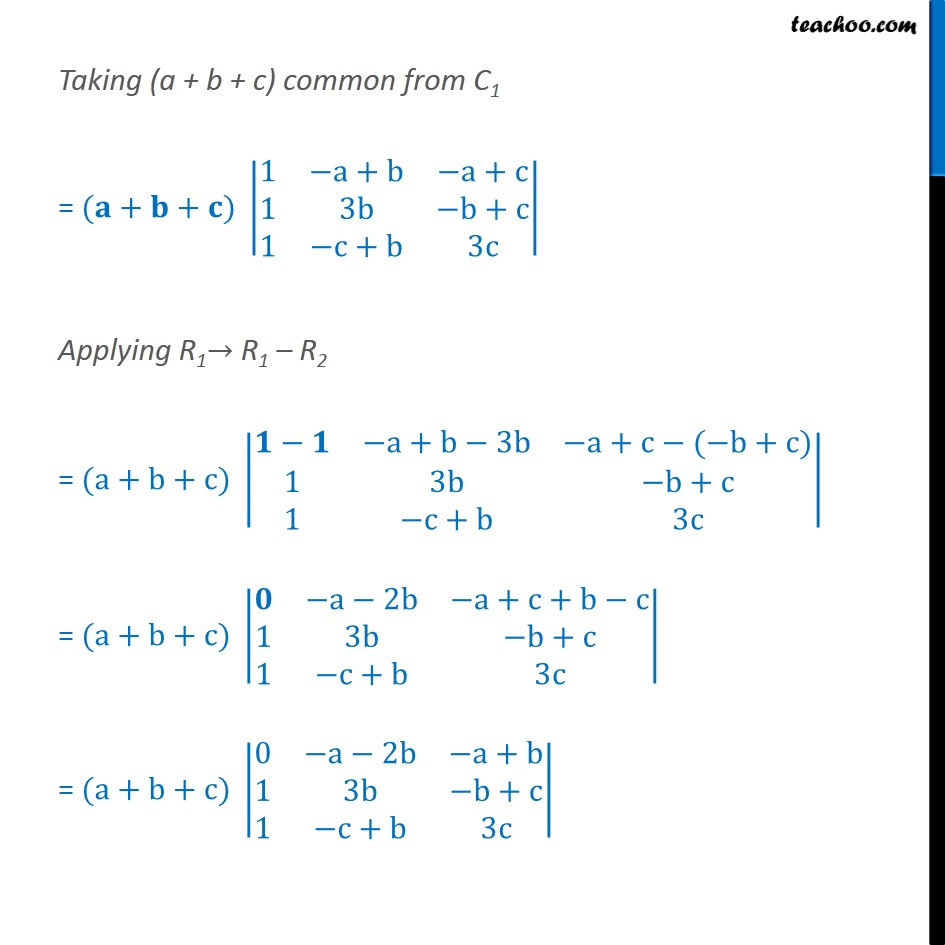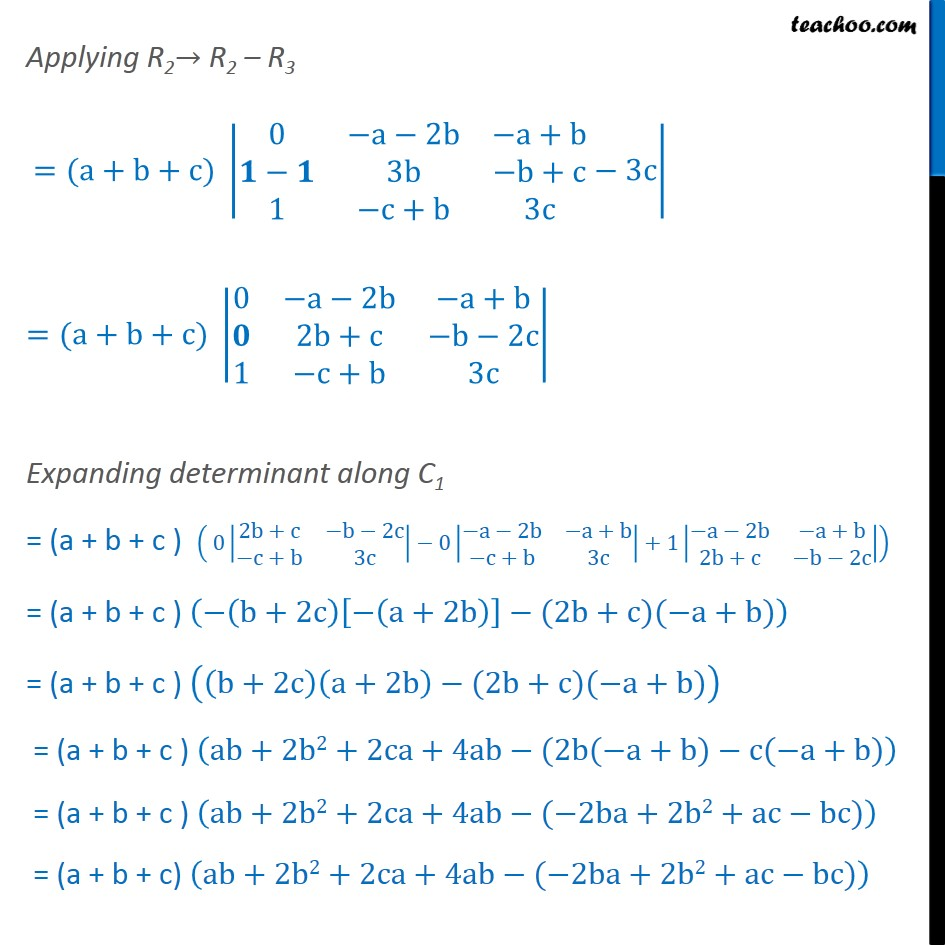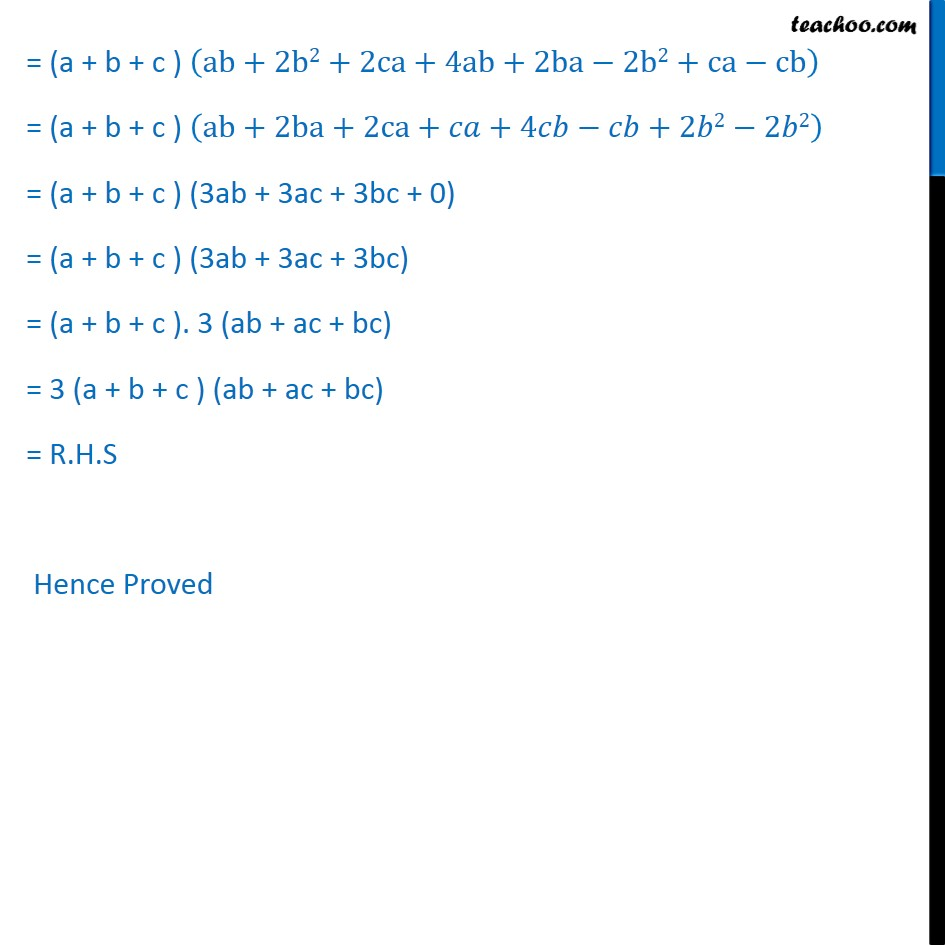Miscellaneous

Chapter 4 Class 12 Determinants
Serial order wiseGet live Maths 1-on-1 Classs - Class 6 to 12

### Transcript

Misc. 13 Using properties of determinants, prove that: 3a a+b a+c b+a 3b b+c c+a c+b 3c = 3 ( a + b + c) (ab + bc + ac) Taking L.H. S 3a a+b a+c b+a 3b b+c c+a c+b 3c Applying C1 C1 + C2 + C3 = 3a a+b a+c a+b a+c b+a 3b b+c 3b b+c c+a c+b 3c c+b 3c = + + a+b a+c + + 3b b+c + + c+b 3c Taking (a + b + c) common from C1 = ( + + ) 1 a+b a+c 1 3b b+c 1 c+b 3c Applying R1 R1 R2 = (a+b+c) a+b 3b a+c ( b+c) 1 3b b+c 1 c+b 3c = (a+b+c) a 2b a+c+b c 1 3b b+c 1 c+b 3c = (a+b+c) 0 a 2b a+b 1 3b b+c 1 c+b 3c Applying R2 R2 R3 =(a+b+c) 0 a 2b a+b 3b b+c 1 c+b 3c 3c =(a+b+c) 0 a 2b a+b 2b+c b 2c 1 c+b 3c Expanding determinant along C1 = (a + b + c ) 0 2b+c b 2c c+b 3c 0 a 2b a+b c+b 3c +1 a 2b a+b 2b+c b 2c = (a + b + c ) b+2c a+2b (2b+c)( a+b) = (a + b + c ) b+2c a+2b (2b+c)( a+b) = (a + b + c ) ab+2b2+2ca+4ab (2b( a+b) c( a+b) = (a + b + c ) ab+2b2+2ca+4ab ( 2ba+2b2+ac bc) = (a + b + c) ab+2b2+2ca+4ab ( 2ba+2b2+ac bc) = (a + b + c ) ab+2b2+2ca+4ab+2ba 2b2+ca cb = (a + b + c ) ab+2ba+2ca+ +4 +2 2 2 2 = (a + b + c ) (3ab + 3ac + 3bc + 0) = (a + b + c ) (3ab + 3ac + 3bc) = (a + b + c ). 3 (ab + ac + bc) = 3 (a + b + c ) (ab + ac + bc) = R.H.S Hence Proved